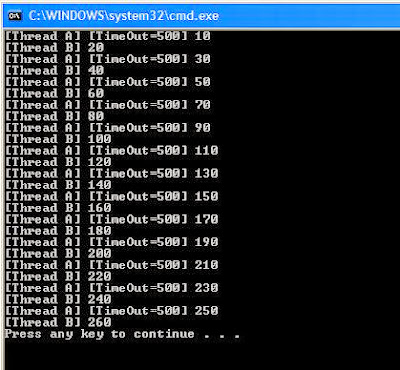## Feb 16, 2011

### Thread Synchronization using Windows Mutex

In my earlier post Thread Synchronization in Windows using Critical Section, I have used Windows Critical Section as Locks for synchronizing the threads. This post talks about using Windows Mutex as synchronization objects and demonstrates it's use with a simple example. Windows functions related with Mutexes are CreateMutex, ReleaseMutex and OpenMutex

```HANDLE CreateMutex(
LPSECURITY_ATTRIBUTES lpMutexAttributes,
BOOL bInitialOwner,
LPCTSTR lpName
);```
```BOOL ReleaseMutex(
HANDLE hMutex
);```
```HANDLE WINAPI OpenMutex(
DWORD dwDesiredAccess,
BOOL bInheritHandle,
LPCTSTR lpName
);```

Key Points

1. A thread acquires a mutex by calling WaitForSingleObject(HANDLE hMutex, DWORD dwMilliseconds);
2. A thread releases mutes by calling ReleaseMutex(HANDLE hMutex);
3. The WaitForSingleObject call returns when the specified object is in the signaled state or when the time out interval has lapsed

The MutexLock class implementation using Windows Mutex synchronization Object
```#ifndef _MutexLock_H_
#define _MutexLock_H_

//File: MutexLock.h

#include <windows.h>
#include <process.h>
#include <iostream>
#include "NonCopyable.h"

namespace examples
{
class MutexLock : public NonCopyable
{
public:
MutexLock()
{
m_hMutex = (HANDLE) ::CreateMutex(0, 0, 0);
if(NULL == m_hMutex)
{
std::cout << "ERROR: Cannot create mutex" << std::endl;
}
}

~MutexLock()
{
::CloseHandle(m_hMutex);
}

void acquire()
{
if(::WaitForSingleObject(m_hMutex, INFINITE) != WAIT_OBJECT_0)
{
std::cout << "ERROR: Cannot acquire mutex" << std::endl;
}
}

bool tryAcquire(size_t timeOut)
{
bool retval = false;
switch(::WaitForSingleObject(m_hMutex, timeOut))
{
case WAIT_OBJECT_0:
retval = true;
break;
case WAIT_TIMEOUT:
std::cout << "ERROR: Cannot acquire mutex" << std::endl;
break;
default:
std::cout << "ERROR: Cannot acquire mutex" << std::endl;
break;
}

return retval;
}

void release()
{
if(::ReleaseMutex(m_hMutex) == 0)
{
std::cout << "ERROR: Cannot release mutex" << std::endl;
}
}

private:
HANDLE m_hMutex;
};

}

#endif //_MutexLock_H_
```

```#ifndef _Noncopyable_H_
#define _Noncopyable_H_

//File: NonCopyable.h

#include <iostream>

namespace examples
{
class NonCopyable
{
protected:
NonCopyable(){}
~NonCopyable(){}

private:
NonCopyable(const NonCopyable&);
NonCopyable& operator=(const NonCopyable&);
};
}

#endif //_Noncopyable_H_
```
Q. When should a time out be used in the call WaitForSingleObject?

A. Your thread should be programmed to return gracefully after the job has been done. Using time out in your code can cause unexpected results which are difficult to identify during debugging.
If a need arises to specify a time out, it's value should be more than the time a thread will spend in the execution of that block of the code

Case 1: The program below uses a random value as the time out. The results as you can see are inconsistent

```#include <windows.h>
#include <process.h>
#include <iostream>
#include <assert.h>
#include "MutexLock.h"

//File: MutexExample.cpp

using namespace examples;

static bool alive = true;
static int current = 0;
static MutexLock lock;

unsigned __stdcall proc1(void *args)
{
int timeOut;
char *name = (char *) args;
while(alive)
{
timeOut = rand() % 1000;
lock.tryAcquire(timeOut);
current = current + 10;
::Sleep(400);
std::cout << "[" << name << "] [TimeOut=" << timeOut << "] " << current << std::endl;
lock.release();
}
return 0;
}

unsigned __stdcall proc2(void *args)
{
int timeOut;
char *name = (char *) args;
while(alive)
{
lock.acquire();
current = current - 10;
::Sleep(400);
std::cout << "[" << name << "] " << current << std::endl;
lock.release();
}
return 0;
}

int main()
{
unsigned t1;
HANDLE h1 = (HANDLE) ::_beginthreadex(0, 0, &proc1, tname1, CREATE_SUSPENDED, &t1);
assert(h1 != 0);

unsigned t2;
HANDLE h2 = (HANDLE) ::_beginthreadex(0, 0, &proc2, tname2, CREATE_SUSPENDED, &t2);
assert(h2 != 0);

::Sleep(10000);
alive = false;
//Wait for threads to terminate gracefully
::WaitForSingleObject(h1, INFINITE);
::WaitForSingleObject(h2, INFINITE);

::CloseHandle(h1);
::CloseHandle(h2);

return 0;
}
```
Sample Run of the above code:Case 2: The proc1 function with an intelligent time out value. The time out value was easy to identify in the example below.

```unsigned __stdcall proc1(void *args)
{
int timeOut;
char *name = (char *) args;
while(alive)
{
timeOut = 500;
lock.tryAcquire(timeOut);
current = current + 10;
::Sleep(400);
std::cout << "[" << name << "] [TimeOut=" << timeOut << "] " << current << std::endl;
lock.release();
}
return 0;
}
```
Sample run after the above change:Case 3: The thread returns gracefully cleaning up all it's resources.

```unsigned __stdcall proc1(void *args)
{
char *name = (char *) args;
while(alive)
{
lock.acquire();
current = current + 10;
::Sleep(400);
std::cout << "[" << name << "] " << current << std::endl;
lock.release();
}
return 0;
}
```
Sample run after the above change: